Math Concepts
Introduction to Lines and Angles
8.8k views

 1 Introduction 2 What are Angles? 3 Angles Sum Property of a Triangle 4 Applications of Lines & Angles 5 Example Problems 6 Advanced Level Problems 7 Learning Outcomes 8 About Cuemath 8 Frequently Asked Questions (FAQs)

28 January 2021

## Introduction

What is a line?

A line is a single-dimensional or one-dimensional figure, with no thickness, and extends in both directions infinitely. Usually, it is called a straight line just to emphasize that there are no curves anywhere in it.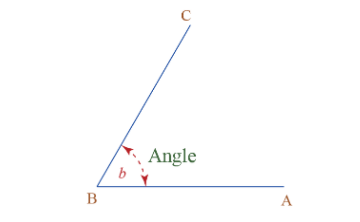In the above picture, AB and BC are two lines meeting at point B. The point B is called the Vertex. The space between the two lines marked as “b” is called the Angle.

Other Definitions included as part of the lines are listed below for immediate reference.

• Line segment: A part of a line with two endpoints is called a line segment. Line segment AB is denoted by AB.
• Ray: A part of a line with one endpoint is called a ray.
• Collinear points and non-collinear points: If three or more than three points are on the same line, then they are called collinear points, otherwise, they are non-collinear points.
• Intersecting Lines and Non-intersecting Lines: Lines PQ and RS are intersecting lines because they are intersecting with each other at O.  Lines AB and CD are non-intersecting (parallel) lines.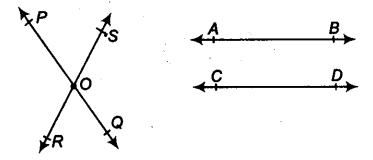Note: The lengths of the common perpendicular at different points on these parallel lines are the same. This equal length is called the distance between two parallel lines.

### Introduction to Lines and Angles-PDF

A line is a single-dimensional or one-dimensional figure, with no thickness, and extends in both directions infinitely. Let us read more about Lines and Angles. Here is a downloadable PDF to explore more.

## What are Angles?

An angle is a space or the gap formed between two lines that meet at a point and the meeting point is called the Vertex. The two lines originating from the same point are called the arms of the angle.

The Angles are classified into different types such as Acute angle, Right angle, Obtuse angle, Straight angle, Reflex angle, and Full angle. These angles are explained in the table given below along with images showing different angles.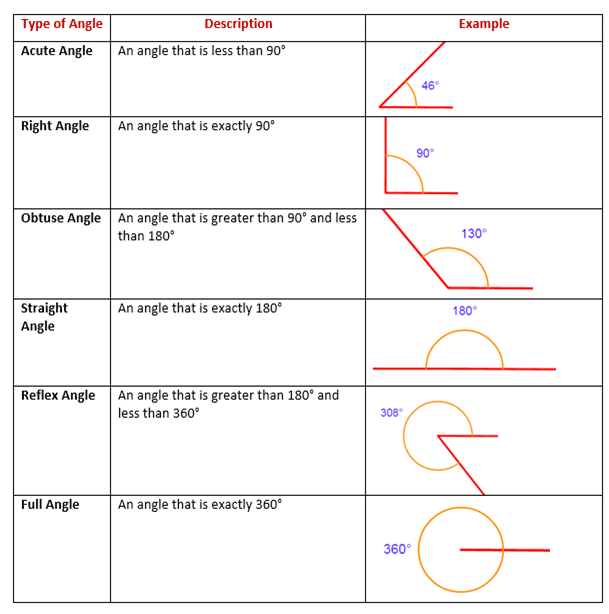When a pair of angles are formed, they are named differently based on a few criteria. The names of the pair of angles and their criteria are given below.

• Complementary Angles: Two angles whose sum is 90° are called complementary angles.
• Supplementary Angles: Two angles whose sum is 180° are called supplementary angles.
• Adjacent Angles: Two angles are adjacent if they have a common vertex, a common arm and their non-common arms are on different sides of the common arm. ∠ABD and ∠DBC are the adjacent angles. Ray BD is their common arm and point B is their common vertex. Ray BA and Ray BC are non-common arms.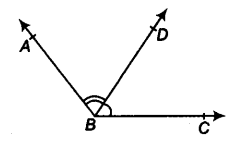Note: ∠ABC = ∠ABD + ∠DBC

• Vertically Opposite Angles: The vertically opposite angles formed when two lines intersect each other at a point. Two lines AB and CD intersect each other at point O, then, there are two pairs of vertically opposite angles. One pair is ∠AOD and ∠BOC and another pair is ∠AOC and ∠BOD.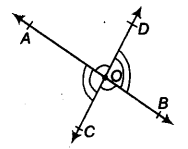If two lines intersect each other, then the vertically opposite angles are equal.  ∠AOD = ∠BOC; ∠COA = ∠DOB

• Linear Pair of Angles: When the sum of two adjacent angles is 180°, then they are called a linear pair of angles.

(i) If a ray stands on a line, then the sum of two adjacent angles so formed is 180°.
(ii) If the sum of two adjacent angles is 180°, then a ray stands on a line (that is the non-common arms form a line). In the above image, Ray OC stands on line AB. ∠AOC + ∠BOC = 180°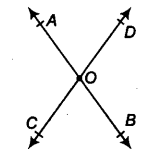• Parallel Lines and a Transversal: A line that intersects two or more lines at distinct points is called a transversal.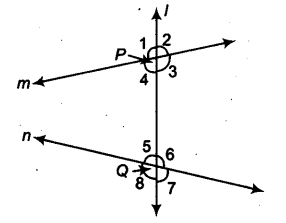Here, line l is a transversal of the lines m and n, respectively.
Line l intersects m and n at P and Q respectively, then four angles are formed at each of the points P and Q namely ∠1, ∠2, ∠3,…, ∠8
∠1, ∠2, ∠7, and ∠8 are called exterior angles.
∠3, ∠4, ∠5, and ∠6 are called interior angles.
We classify these eight angles into the following groups:
1. Corresponding angles
∠1 and ∠5
∠2 and ∠6
∠4 and ∠8
∠3 and ∠7

2. Alternate interior angles
∠4 and ∠6
∠3 and ∠5

3. Alternate exterior angles
∠1 and ∠7
∠2 and ∠8

4. Co-interior angles - Interior angles on the same side of the transversal
∠4 and ∠5
∠3 and ∠6
Note: Interior angles on the same side of the transversal are also referred to as consecutive interior angles or allied angles or co-interior angles.

Relation Between the Angles when Line m is Parallel to Line n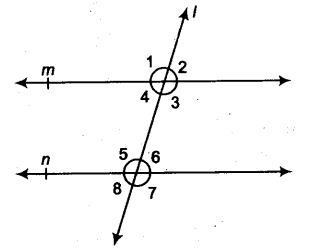(i) If a transversal intersects two parallel lines, then each pair of corresponding angles is equal. i.e., ∠1 = ∠5, ∠2 = ∠6 , ∠4 = ∠8, and ∠3 = ∠7

(ii) If a transversal intersects two lines such that a pair of corresponding angles are equal, then the two lines are parallel to each other.

(iii) If a transversal intersects two parallel lines, then each pair of alternate interior angles is equal. i.e., ∠4 = ∠6 and ∠3 = ∠5

(iv) If a transversal intersects two lines such that a pair of alternate interior angles are equal, then the two lines are parallel.

(v) If a transversal intersects two parallel lines, then each pair of interior angles on the same side of the transversal is supplementary. i.e., ∠4 + ∠5 = 180° and ∠3 + ∠6 = 180°

(vi) If a transversal intersects two lines such that a pair of interior angles on the same side of the transversal is supplementary, then the two lines are parallel.

Lines Parallel to the Same Line: If two lines are parallel to the same line, will they be parallel to each other.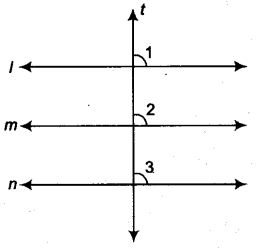## Angles Sum Property of a Triangle

1. The sum of the angles of the triangle is 180°.
∠1 + ∠2 + ∠3 = 180°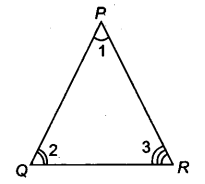2. If a side of a triangle is produced, then the exterior angle, formed is equal to the sum of the two interior opposite angles.

∠4 = ∠1 + ∠2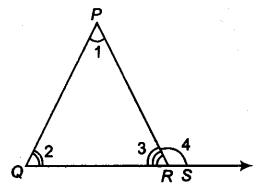Note: An exterior angle of a triangle is greater than either of its interior opposite angles.

## Applications of Lines & Angles

Angles are used in daily life. Civil Engineers and Architects use angles for building infrastructures such as roads, construction work, animations, bearing tools, crossroads, etc. Angles are also used by carpenters to make chairs, tables, cots, and sofas. Angles are also used by Artists in, their creations such as portraits and paintings.

Some examples of Angles in daily life.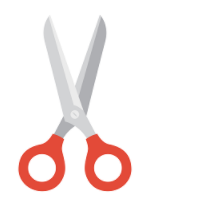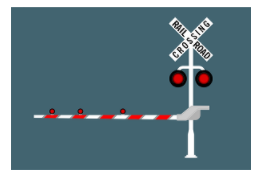## Example Problems

Question 1: In the angles of a triangle are in the ratio 2:3:4, then the triangle formed will be
(A) Right-angled triangle         (B) Isosceles triangle
(C) Scalene triangle                 (D) Obtuse angled triangle

Answer: We know that the sum of the angles of a triangle is 180°.
Here in the question, we are provided with the ratio of the three angles. If we take the value as “x” the ratio becomes, 2x:3x:4x.

As per the angle sum property,
$$2x + 3x + 4x = 180°$$
$$9x = 180°$$
$$x = 180 ÷ 9 = 20$$

Substituting the value of “$$x$$" in the ratio, the ration becomes
$$(2*20):(3*20):(4*20) = 40:60:80$$

Since all the three angles are different and as per the given options, the correct answer to this question is (C).

 (C) Scalene triangle

Question 2: In the given figure, find the value of ∠EOD.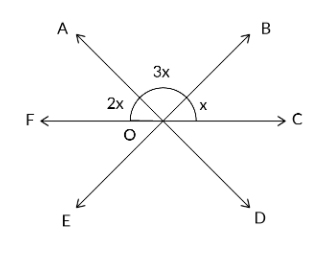(A) 25°                        (B) 70°
(C) 80°                        (D) 90°

Answer: FC is a straight line. Therefore the sum of all the angles on either side of the straight line is equal to 180°.

Here in the image given, we need to find ∠EOD.
∠EOD is the vertically opposite angle of ∠AOB.
∠AOB is given as $$3x$$. For that, we need to find the value of $$x$$
∠FOA+∠AOB+∠BOC=180°
$$2x + 3x + x = 180°$$
$$6x = 180°$$
$$x = 180 ÷ 6 = 30°$$
Now substituting the value of x in the ∠AOB = $$3x = 3*30 = 90°$$
$$∠AOB = 90°$$
Therefore, $$∠EOD = 90°$$ (Vertically opposite angles) of option, D is the correct answer.

 (D) 90°

Question 3: In the given figure, if CE∥BA, then the value of ∠ACB is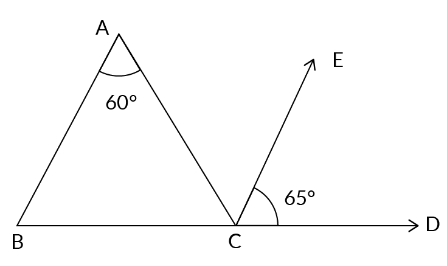(A) 60°                        (B) 55°
(C) 70°                        (D) 90°

Given: CE is parallel to BA
Since CE II BA, BD has become the transversal.

Therefore, ∠ABC and ∠ECD are corresponding angles. We know that corresponding angles on the same side of the transversal are the same. So these two angles are equal.
$$∠ECD = 65° = ∠ABC$$

By the angle sum property, the sum of three angles of a triangle = 180°

Here in the △ABC
$$∠ABC + ∠BAC + ∠ACD = 180°$$
Substituting the values of ∠ABC and ∠BAC in the above equation, we could find the value of ∠ACD at 55°.  The correct answer is option B.

 (B) 55°

Question 4: The angles which differ by 38° and are complementary to each other, are

(A) 38°, 52°                            (B) 71°, 109°
(C) 26°, 154°                          (D) 64°, 26°

Answer: The sum of the complementary angles is (always) 90°. Let the smallest angle be represented by $$x$$
Then we get the following sum of angles.
$$x +(x+38) = x +x +38 = 2x+38$$
This sum equals $$90°$$, so we get
$$2x + 38 = 90$$
$$2x = 52$$
$$x = 52 ÷ 2 = 26$$

Now we know that the smallest angle measures 26°. The bigger angle measures$$x + 38$$, so
$$x + 38 =26+38 = 64$$
Hence, the measure of the angles are $$26°$$ and $$64°$$

Therefore, the correct answer is option D.

 (D) 64°, 26

Question 5: The value of x, in the given triangle, is _____.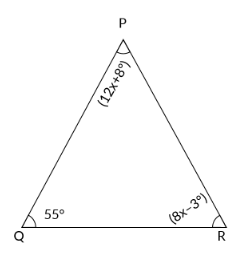(A) 4°                                (B) 5°
(C) 6°                                (D) 8°

By Angle sum property, $$∠P + ∠Q + ∠ R = 180°$$
$$12x+ 8 + 55 + 8x - 3 = 180°$$
$$12x + 8x + 8 + 55 - 3 =180°$$
$$20x + 63 - 3 = 180°$$
$$20x + 60 = 180°$$
$$20x = 180° - 60°$$
$$x = 120 ÷ 20$$
$$x= 6$$
Therefore the correct answer is option C.

 (C) 6°

Question 1: In the given figure, if OCD is an isosceles triangle in which OD and OC are equal, then what will be the value of ∠OCD?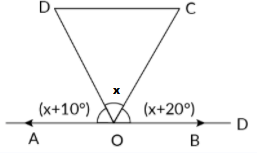(A) 70°                                    (B) 50°
(C) 65°                                    (D) 45°

$$x + 10° + x + x +20°= 180°$$
$$3x +30° = 180°$$
$$3x = 150°$$
$$x = 50°$$
∠OCD = ∠ODC   [∵OD = OC]
Now,
$$∠OCD + ∠ODC + x = 180°$$
$$∠OCD + ∠OCD + 50° = 180°$$
$$2∠OCD = 130°$$
$$∠OCD = 65°$$
Therefore the correct answer is (C) 65°.

 (C) 65°

Question 2: In the given figure, AB∥DC. Then the value of x is _______.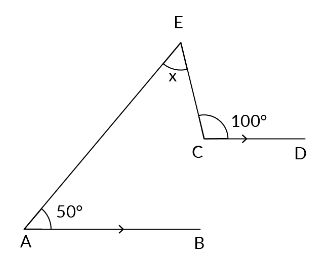(A) 25°                                (B) 30°
(C) 45°                                (D) 50°

Answer: Given AB II CD, extending the line CD to meet the line AE at F, as shown in the below figure.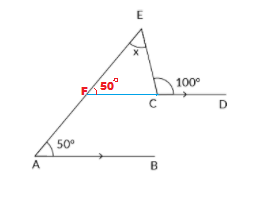∠EAB and ∠EFC are corresponding angles. Therefore ∠EAB = ∠EFC = 50°
Now, in △AFC, the exterior angle at C is 100°
We know that the exterior angle is equal to the sum of two opposite angles in the triangle.
Therefore,
∠EFC+∠FEC = ∠ECD
$$50°+x=100°$$
$$x=100 - 50 = 50°$$
The correct answer is option D.

 (D) 50°

## Learning Outcomes

• Identify lines, line segments, rays, and angles.
• Classify angles as acute, right, obtuse, or straight.
• Identify complementary and supplementary angles.
• Find measures of angles.

For more details you can refer to the blogs from the below links:

Cuemath, a student-friendly mathematics platform, conducts regular Online Live Classes for academics and skill-development, and their Mental Math App, on both iOS and Android, is a one-stop solution for kids to develop multiple skills. Understand the Cuemath Fee structure and sign up for a free trial.